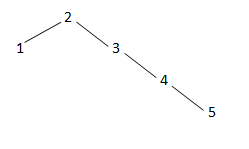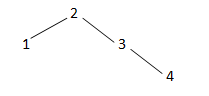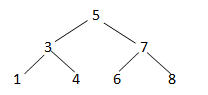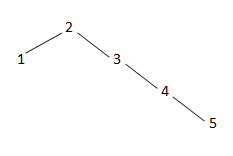# C++ program to check whether a given Binary Search Tree is balanced or not?

Here, we are going to implement a C++ program that will check whether a given binary search tree is a balanced tree or not?
Submitted by Bhanu Pratap Raghav, on December 10, 2018

Description: Solution to check the given Binary Search tree is balanced or not.

Problem Statement: Write a program that accepts input from user to form a binary search tree and check whether the formed tree is balanced or not.

Example 1:

Input: 2 1 3 4 5 -1Output: Given BST is Balanced : False

Example 2:

Input: 2 1 3 4 -1Output: Given BST is Balanced : True

Explanation...

What do you mean by height of a tree?

A height of a tree is the largest number of edges in a path from node to a leaf node. If a tree has only one node: i.e. root node itself then the height of tree is 0.

Example:Height of tree: 2 ( maximum 2 edges from root to leaf)

Check when tree is balanced:

A non-empty binary search tree is said to be balanced if:

1. Its left subtree is balanced.
2. Its Right subtree is balanced.
3. The difference between heights of left and right subtree is less than or equal to 1.

Example:At root height of left subtree is 1 , whereas height of right subtree is 3

Difference = 3-1=2 (which is greater than 1) i.e. Tree is not balanced.

Algorithm:

1. Set root=root of given binary search tree.
2. Set leftHt= height of left subtree.
3. Set rightHt= height of right subtree.
4. if abs(leftHt – rightHt ) > 1
return false;
else isHeightBalanced(root->left) &&isHeightBalanced(root->right)

C++ Code

```#include <iostream>
#include <cmath>

using namespace std;

class TreeNode {
public:
int data;
TreeNode* left;
TreeNode* right;
TreeNode(int d) {
data = d;
left = NULL;
right = NULL;
}
};

TreeNode* insertInBST(TreeNode* root, int x) {
if (root == NULL) {
TreeNode* tmp = new TreeNode(x);
return tmp;
}

if (x < root->data) {
root->left = insertInBST(root->left , x);
return root;
} else {
root->right = insertInBST(root->right, x);
return root;
}
}

TreeNode* createBST() {
TreeNode* root = NULL;
int x;
cin >> x;

//Take input until user inputs -1
while (true) {
if (x == -1) break;
root = insertInBST(root, x);
cin >> x;
}
return root;
}

//Calculate height of tree with given root
int height(TreeNode* root) {
if (root == NULL) return 0;

int leftHt = height(root->left);
int rightHt = height(root->right);
int curHt = max(leftHt, rightHt) + 1;
return curHt;
}

//Check whether tree is balanced or not
bool isHeightBalanced(TreeNode* root) {
if (!root) {
return true;
}

int leftHt = height(root->left);
int rightHt = height(root->right);

if (abs(leftHt - rightHt) > 1)
return false;
return isHeightBalanced(root->left) && isHeightBalanced(root->right);
}

int main() {
//Input BST
cout<<"Input Tree elements : ";
TreeNode* root = createBST();

cout << "Given BST is Balanced : ";
if (isHeightBalanced(root)) {
cout << "True";
}
else {
cout << "False";
}

return 0;
}
```

Output

```First run:
Input Tree elements(stop taking input when  -1 encountered) : 2 1 3 4 5 -1
Given BST is Balanced : False

Second run:
Input Tree elements(stop taking input when  -1 encountered) : 2 1 3 4  -1
Given BST is Balanced : True
```

Preparation

What's New

Top Interview Coding Problems/Challenges!